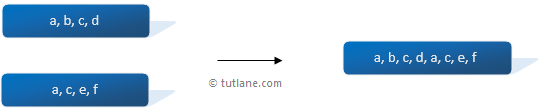# LINQ Concat Method

In LINQ, the Concat method or operator is used to concatenate or append two collection elements into a single collection, and it does not remove duplicates from the two sequences.

Following is the pictorial representation of the LINQ Concat method.In the above diagram, two list elements are combined into a single collection.

## Syntax of LINQ Concat Method

Following is the syntax of using the LINQ Concat method to combine two sequences into a single sequence.

C# Code

var result = arr1.Concat(arr2);

VB.NET Code

Dim result = arr1.Concat(arr2)

If you observe the above syntax, we are concatenating two lists into a single list.

## Example of LINQ Concat Method

Following is an example of the LINQ Concat method.

C# Code

using System;
using System.Linq;

namespace Linqtutorials
{
class Program
{
static void Main(string[] args)
{
string[] arr1 = { "a", "b", "c", "d" };
string[] arr2 = { "c", "d", "e", "f" };
var result = arr1.Concat(arr2);
foreach (var item in result)
{
Console.WriteLine(item);
}
}
}
}

VB.NET Code

Module Module1

Sub Main()
Dim arr1 As String() = {"a", "b", "c", "d"}
Dim arr2 As String() = {"c", "d", "e", "f"}
Dim result = arr1.Concat(arr2)
For Each item In result
Console.WriteLine(item)
Next
End Sub
End Module

If you observe the above example, we are concatenating two sequences, “arr1” and “arr2” into a single sequence using the LINQ Concat method.

## Result of LINQ Contact Method Example

Following is the result of the LINQ Concat method example.

a
b
c
d
c
d
e
f

This is how we can use LINQ concat method to combine multiple sequences into a single sequence in c#, vb.net.